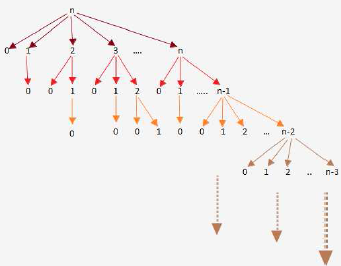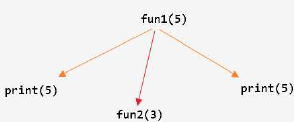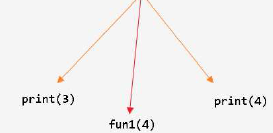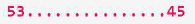Courses

# Recursion MCQ - 1

## 10 Questions MCQ Test GATE Computer Science Engineering(CSE) 2022 Mock Test Series | Recursion MCQ - 1

Description
This mock test of Recursion MCQ - 1 for Computer Science Engineering (CSE) helps you for every Computer Science Engineering (CSE) entrance exam. This contains 10 Multiple Choice Questions for Computer Science Engineering (CSE) Recursion MCQ - 1 (mcq) to study with solutions a complete question bank. The solved questions answers in this Recursion MCQ - 1 quiz give you a good mix of easy questions and tough questions. Computer Science Engineering (CSE) students definitely take this Recursion MCQ - 1 exercise for a better result in the exam. You can find other Recursion MCQ - 1 extra questions, long questions & short questions for Computer Science Engineering (CSE) on EduRev as well by searching above.
QUESTION: 1

### What will be the output of the following C program? void count (int n) { static int d=1; printf ("%d",n); printf ("%d",d);  d++; if (n>1) count (n-1);  printf ("%d",d); } void main(){  count (3); }

Solution:

Here d is Static, so the value of d will persists between the function calls.

1. count(3) will print the value of n and d and increments d and call count(2) = prints 3 1.
2. count(2) will print the value of n and d and increments d and call count(1) = prints 2 2.
3. count(1) will print the value of n and d and increments d => prints 1 3.

Now it will return and prints the final incremented value of d which is 4, 3 times.
So, option A is correct = 3 1 2 2 1 3 4 4 4

QUESTION: 2

### A language with string manipulation facilities uses the following operations head(s): first character of a string tail(s): all but exclude the first character of a string concat(s1, s2): s1s2 For the string "acbc" what will be the output of concat(head(s), head(tail(tail(s))))

Solution:

concat(a,head(tail(tail(acbc))))
concat(a,head(tail(cbc)))
concat(a,head(bc))
concat(a,b)
ab.

QUESTION: 3

### Consider the following C function: int f(int n) { static int i = 1; if(n >= 5) return n; n = n+i;     i++; return f(n); } The value returned by f(1) is

Solution:

answer is 7.as,
f(1):n=2,i=2
f(2):n=4,i=3
f(4):n=7,i=4
f(7):print(n)===>>> 7<ans>

QUESTION: 4

double foo(int n)

{

int i;

double sum;

if(n == 0)

{

return 1.0;
}

else

{

sum = 0.0;

for(i = 0; i < n; i++)

{

sum += foo(i);

}
return sum;

}
}

The space complexity of the above code is?

Solution:A. The code here is storing only local variables. So, the space complexity will be the recursion depth- maximum happening for the last iteration of the for loop- foo(n-1) - foo(n-2) - .... - foo(0) all live giving space complexity O(n).

B. To store the n values we need space complexity O(n). So, the space complexity won't change and will be O(n).

QUESTION: 5

double foo(int n)

{

int i;

double sum;

if(n == 0)

{return 1.0;

}

else

{

sum = 0.0;

for(i = 0; i < n; i++)

{

sum += foo(i);

}

return sum;

}
}

Suppose we modify the above function foo() and stores the value of foo(i) 0 <= i < n, as and when they are computed. With this modification the time complexity for function foo() is significantly reduced. The space complexity of the modified function would be:

Solution:

The modification being proposed is tabulation method of Dynamic Programming.
So let we have an auxillary array which stores the value of foo(i) once it is computed and can be used at later stage .So foo(i) is not called again , instead lookup from the table takes place. It would be something like :

#include <stdio.h>
#define max 1000
int foo[max];

foo(int n) {
if(foo[n] == -1) //compute it and store at foo[n] and return
else // return foo[n] directly
}

int main(void) {
//initialize all elements of foo[max] to -1
return 0;
}Using this approach , we need an auxillary array of size n to store foo(i) where i ranges from 0 to n-1.

So space complexity of this method = O(n) And function foo(n) computes 2n - 1

QUESTION: 6

int f(int n)

static int r = 0;

if (n <= 0) return1;

if (n > 3)

{   r = n;

return f(n-2) + 2;

}
return f(n-1) + r;

}

What is the value of f(5)?

Solution:

f(5) = 18.
f(3) + 2 = 16 + 2 = 18
f(2) + 5 = 11 + 5 = 16

f(1) + 5 = 6 + 5 = 11
f(0) + 5 = 1+5 = 6
Consider from last to first. Since it is recursive function.

QUESTION: 7

The function f is defined as follows:

int f (int n) {

if (n <= 1) return 1;

else if (n % 2 == 0) return f(n/2);

else return f(3n - 1);
}

Assuming that arbitrarily large integers can be passed as a parameter to the function, consider the following statements.

i. The function f terminates for finitely many different values of n ≥ 1.

ii. The function f terminates for infinitely many different values of n ≥ 1.

iii. The function f does not terminate for finitely many different values of n ≥ 1.

iv. The function f does not terminate for infinitely many different values of n ≥ 1.

Which one of the following options is true of the above?

A. i and iii

B. i and iv

C. ii and iii

D. ii and iv

Solution:

The function terminates for all powers of 2 (which is infinite), hence (i) is false and (ii) is TRUE.
Let n = 5.
Now, recursive calls will go like 5 - 14 - 7 - 20 - 10 - 5 -
And this goes into infinite recursion. And if we multiply 5 with any power of 2, also result will be infinite recursion. Since, there are infinite powers of 2 possible, there are infinite recursions possible (even considering this case only). So, (iv) is TRUE and (iii) is false.
So, correct answer is (D)

QUESTION: 8

Consider the following two functions.

void fun1(int n) {

if(n == 0) return;

printf("%d", n);

fun2(n - 2);

printf("%d", n);

}

void fun2(int n) {

if(n == 0) return;

printf("%d", n);

fun1(++n);

printf("%d", n);

}

The output printed when fun1 (5) is called is

Solution:• Unroll recursion up to a point where we can distinguish the given options and choose the correct one!
• Options B and D are eliminated.
• A is the answer.
QUESTION: 9

Consider the following computation rules. Parallel-outermost rule: Replace all the outermost occurrences of F (i.e., all occurrences of F which do not occur as arguments of other F's) simultaneously. Parallel - innermost rule: Replace all the innermost occurrences of F (i.e.,all occurrences of F with all arguments free of F's) simultaneously. Now consider the evaluations of the recursive program over the integers.

F(x, y) <== if x = 0 then 0 else

[ F(x + 1, F(x, y)) * F(x - 1, F(x, y))]

where the multiplication functions * is extended as follows:

0 * w & w * 0 are 0

a * w & w * a are w (for any non-zero integer a)

w * w is w

We say that F(x, y) = w when the evaluation of F(x, y) does not terminate. Computing F(1, 0) using the parallel - innermost and parallel - outermost rule yields

Solution:

Answer is A) w and 0

If we solve using parallel innermost rule
F(1,0) = F(2,F(1,0)) * F(0,F(1,0))

=F(2, F(2,F(1,0)) * F(0,F(1,0)) ) * F(0, F(2,F(1,0)) * F(0,F(1,0)) )

Since computation of F(1,0) goes on
we assign F(1,0) to w
So F(1,0)= w

Using parallel outermost rule
F(1,0)= F(2,F(1,0)) * F(0,F(1,0))
= F(2,F(1,0)) * 0
= 0

QUESTION: 10

Consider the class of recursive and iterative programs. Which of the following is false?

Solution:

Computable function: those which can be incorporated in a program using for/while loops.

Total Function: Defined for all possible inputs
Well Defined: if its definition assigns it a unique value.

It was a belief in early 1900s that every Computable function was also Primitively Recursive. But the discovery of Ackermann function provided a counter to it.
The class of primitive recursive functions is a small subclass of the class of recursive functions. This means that there are some functions which are Well-Defined Total Functions and are Computable BUT Not primitively recursive; eg. Ackermann function.

This makes all options from option A to option D as True.
But option E as
. As iterative programs are equivalent to only Primitively Recursive class.